# An Experimental Study on Silicon Carbide-water Nanofluids for Heat Transfer Applications

An Experimental Study on Silicon Carbide-water Nanofluids for Heat Transfer Applications

Sneha PonnadaTunuguntla Subrahmanyam Sureddy Vishveshwara Naidu

Centre for Nanotechnology, AUCE (A), Andhra University, Visakhapatnam 530003, India

Department of Mechanical Engineering, AUCE (A), Andhra University, Visakhapatnam 530003, India

Department of Chemical Engineering, AUCE (A), Andhra University, Visakhapatnam 530003, India

Corresponding Author Email:
p.sneha@ymail.com
Page:
497-503
|
DOI:
https://doi.org/10.18280/ijht.370216
8 May 2018
|
Accepted:
20 June 2019
|
Published:
30 June 2019
| Citation

OPEN ACCESS

Abstract:

The effect of perforated twisted tapes with alternate axis (PATT), perforated twisted tapes (PTT) and regular twisted tapes (TT) are compared by an experimental investigation in a circular tube. The testing fluid employed for circulation in the tube is Silicon carbide (SiC)-water nanofluid and the measurements are done under constant heat flux condition. The PATT with different twist ratios are also studied. Experiments are conducted within the Reynolds number range of 3000 – 16000 for the SiC nanofluid, containing SiC nanoparticles obtained after 5 hrs of ball milling with weight concentration of 0.1 %. The heat transfer rates in the tube fitted with TT, PTT and PATT at TR = 3 are respectively improved up to 9.59 %, 18 % and 19.51 % of that in the plain tube. Similarly, the increase in friction factor for TT, PTT and PATT at a TR=3 is 22.5 %, 24 % and 28.9 % respectively, compared to that of the plain tube. As PATT is giving maximum heat transfer enhancement, the empirical correlations were established for its Nusselt number and friction factor at different twist ratios and the obtained correlations are fitted with the experimental data. Hence, the passive elements when employed along with the nanofluid gives better energy efficiency along with lower operating cost and is therefore suggested for commercial heat transfer applications.

Keywords:

friction factor, heat transfer, Nusselt number, SiC nanofluid, twisted tapes, twist ratio

1. Introduction

The heat transfer enhancement of the fluids has been developed and applied for many heat exchange systems. There are many ways in which the heat transfer can be enhanced and among them, the use of nanofluids and the use of passive elements in the heat pipes and heat exchangers are of prime importance as they have reported a reasonable improvement in the heat transfer coefficient.

Nanofluids are the fluids containing nanoparticles of higher thermal conductivity and they reported a greater enhancement in the thermal properties when compared to the conventional heat transfer fluids like water, ethylene glycol and a combination of both. The heat transfer rates obtained by the different combinations of nanoparticles and the base fluids are being studied by many researchers. Hence, the conventional fluids for cooling and heating purposes are replaced by many newer fluids. Among the various nanoparticles, Silicon Carbide (SiC) is one of the promising materials employed to fabricate effective nanofluids as SiC is easily available and has a higher thermal conductivity. Not much research is done on the SiC/water for analysing the heat transfer coefficient of the nanofluid. Yu et al., have reported results for 3.7 % concentration of 170 nm SiC particles suspended in water at constant Reynolds number . Timofeeva et al., have stated that the use of a SiC/water nanofluid will be beneficial in the laminar flow regime when particles are larger than ∼50 nm, while for turbulent flow average particle sizes should be larger than∼90 nm . In their later work, Timofeeva et al., have also reported investigations on SiC/water nanofluid over the base fluid with 90 nm SiC particle at 4 vol.% . Nader et al. have stated results for the enhancement in the heat transfer coefficient at constant Reynolds number for SiC at 9 wt. % in water-ethylene glycol base fluid .

The researchers have investigated on many passive elements i.e., inserts and have reported their investigations. Among them, different types of twisted tapes are employed and studied as they are easy manufacture due to their non-complex configuration and will result in a relatively lower pressure drop. For the heat transfer improvement some modifications are done on the conventional tapes. Farhadi et. al. found that counter-swirl twisted tapes have greater Nusselt number enhancement ratios and friction factors when compared to co-swirl twisted tapes and also did a review study on TT for turbulent flows . The butterfly inserts with different inclined angles in a heat exchanger are considered in the study of Shabanian et al. . Eiamsa-ard et al., have reported on multiple TT vortex generators , full-length dual TT elements  in tandem at y/w =3.0, 4.0 and 5.0, regularly-spaced dual TT, the oblique delta winglet TT and straight delta winglet TT , the short length twisted tape inserts and full-length tapes , TT consisting of centre wings and alternate axis , serrated TT  and peripherally-cut TT . Eiamsa-ard et al., then stated results for the peripherally-cut TT , the peripherally cut TT with alternate axis. Eiamsa-ard et al., also examined alternate clockwise and counter-clockwise TT . The trapezoidal cut TT in a double pipe U-tube heat exchanger was investigated by Prasad et al. . The thermal analysis of fluid flows and different approaches for applications in thermal engineering are studied by Kashif Ali Abro et al. [17-20].

## f1.pngFigure 1. XRD patterns of the particles obtained after different times of ball milling

There is a modification of the conventional twisted tape inserts, with the use of a heat pipe as a heat transport device operating in the flow regime with Reynolds number range of 3000-16000 in the current work. The thermal performance of water in a circular tube with twisted tapes, perforated twisted tapes and perforated twisted tapes with alternate axis is reported by Ponnada et al. . And the enhancement of heat transfer and friction factor of SiC nanofluid at 0.1 wt. % concentration containing particles ball milled after 5 h is circulated in a plain tube containing twisted tapes, perforated twisted tapes and perforated twisted tapes with alternate axis are then compared.

2. Characterization and Thermo-physical Properties of the Nanofluid

A detailed study on the thermal phenomena of the nanofluids along with the characterization of Silicon carbide (SiC) nanoparticles before and after dispersing in distilled water (DIW) is done. The pH of the fluids is adjusted to be between 9 – 10 before and after sonication. The sonication of the nanofluid is done by using a probe sonicator.

The stability of the suspensions is measured and analysed. The crystallite size of SiC particles obtained after 5 h, 10 h and 15 h ball milling are 27 nm, 39 nm and 62 nm respectively from XRD analysis. The particles are mostly indexed for a hexagonal crystal structure. The powder XRD patterns of the SiC nanoparticles at different ball milled times shown in the Figure 1. The nanofluids are prepared at different concentrations of 0.04 wt. %, 0.08 wt. % and 0.1 wt. % as they have reported a better stability from the zeta potential analysis and a relatively lesser particle size from dynamic light scattering (DLS) results. The stability of the nanofluids is measured from a zetasizer Nano ZS90. The visual inspection technique is employed to study the sedimentation behavior of the nanofluids. The SiC nanofluids are then tested for thermal conductivity (TC) in a KD2Pro thermal conductivity analyzer and viscosity in a Physica MCR 51 rheometer.

The maximum increase in thermal conductivity (TC) and viscosity of the nanofluids with that of the base fluid at 0.1 wt. % is 19 % and 94 % respectively at 30 oC is observed for the particles ball milled for 5 h. The density and specific heat of the SiC nanofluids are determined from the mixture equations.

The thermo-physical properties of SiC nanoparticles with crystalline size of 62 nm and SiC-water nanofluid with a weight concentration of 0.1 % are tabulated in the Table 1.

Table 1. Thermophysical properties

 Fluid/ nanoparticles Density (in kg/m3) Specific heat (in J/kg-K) Thermal Conductivity (in W/m-K) SiC nanoparticles 3220 511.6 120 Base fluid (water) 1000 4185.5 0.6 SiC-water nanofluid 1222 3818.11 0.714
3. Technical Details of Tapes

The aluminium twisted tapes used in the work are of 12.5 mm width (D) and 0.8 mm thickness (δ). The twisted tapes (TT) with different twist ratios (TR) i.e., p/D = 3, 4, 5 are made by twisting the aluminium strips of above-mentioned dimensions; where p is the pitch as shown in the Figure 2. Some of the other aluminium strips are perforated at every 12.5 mm distance on the strip. The perforated strips are again twisted to obtain perforated twisted tapes (PTT). The twisted perforated strips are again bent to 90o to obtain an alternate axis for every 100 mm distance for different twist ratios (TR) i.e., p/D=3, 4, 5. The twisted tape (TT), the perforated twisted tape (PTT) and the perforated twisted tape with alternate axis (PATT) are shown in the Figure 3. The pitch (p) and the width (D) are depicted in the Figure 4. showing PTT and PATT.

## f2.jpgFigure 2. Twisted tapes with TR=3, 4 and 5

## f3.jpg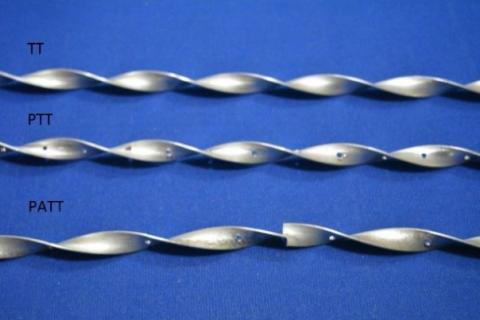Figure 3. TT, PTT and PATT

## f4.jpg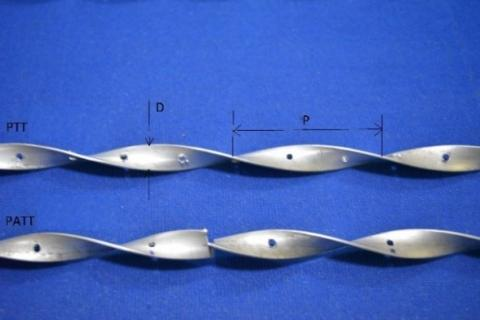Figure 4. PTT and PATT indicating pitch (p) and width (D)

4. Test Facility

The copper tube in the test section is of 2600 mm in length with outer diameter of 31.8 mm and inner diameter of 28.6 mm. A Nichrome heating wire of 21-gauge and resistance of 46 Ohms is wound all over the length of the tube. The heater wire is wound with ceramic beads, which acts as electrical insulation. A constant heat flux condition is considered in the present work. The schematic representation of the experimental setup used for the present study is shown in the Figure 5.

## f5.png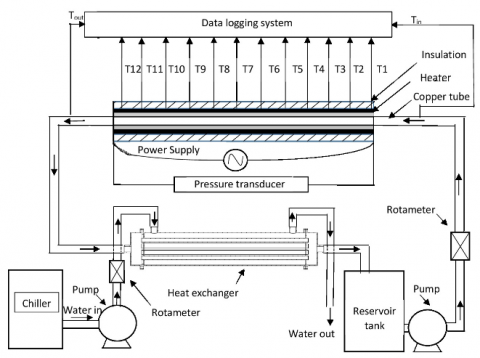Figure 5. Schematic representation of the experimental setup

The outer surface of the tube is brazed with twelve K-type thermocouples all along the circumference of the circular tube. Two RTD PT 100 type temperature sensors are used to measure the inlet and outlet temperatures of the tube. The asbestos and fiber glass insulation are provided over the copper pipe, to reduce the heat loss. A pressure transducer is placed to measure pressure drop along the pipe. The data acquisition (DAQ) system is connected to the test section to display temperatures, pressures, voltage and amperage. A program is written in the lab view software where all values are inputted from DAQ at each flow rate to obtain Nusselt number and heat transfer coefficient from the temperature readings. Similarly, the friction factor is obtained from the pressure drop readings.

A shell and tube heat exchanger are employed to cool the fluid coming out of the test section, using the cool water circulated through the chiller unit. The cooled fluid is again collected back in the reservoir tank and is recirculated. The flow rates at the pipe section and the heat exchanger are regulated by two rotameters with bypass valves. The rotameter on the pipe section side can measure flow rates in the range 300 L/h to 1200 L/h. A 0.5 HP pump is used to pump the fluid from the reservoir tank to the entrance of the test section.

5. Heat Transfer and Friction Factor Calculations

At steady state, according to the heat balance, the heat loss was found to be in between 5 % and 10 % of the electrical power input.

The heat transfer coefficient (h) is calculated from the following equation:

$h=m c_{p}\left(T_{o}-T_{i}\right) /\left(T_{S}-T_{B}\right)$ (1)

where, $T_{B}=\frac{\left(T_{o}+T_{i}\right)}{2}$

An average of the twelve wall temperatures considered all along the pipe and $T_{S}=\left(T_{1}+T_{2}+\cdots T_{12}\right) / 12$

The heat transfer rate in terms of Nusselt number $(N u)$ can be written as,

$N u=h D / k$ (2)

The friction factor is calculated from pressure drop $(\Delta p)$ across the tube length and is defined as,

$f=2 \Delta p D / U^{2} L$ (3)

6. Experimental Results and Discussion

6.1 Validation tests on the base fluid in the plain tube

Under constant heat flux condition, the base fluid DIW is run in the test section at Reynolds number range of 3000 – 16000. Thereby, the temperature and pressures are recorded for calculating the Nusselt number and friction factor. Hence, to test the reliability of the test section, the obtained values are compared with the theoretical correlations available from the literature as shown in the Figure 6.

Dittus-Boelter equation (4) is employed to compare the Nusselt number as given below i.e.,

Dittus-Boelter correlation:

$N u=0.023 R e^{0.8} P r^{0.3}$ (4)

Whereas, Blasius equation (5) is used to compare the friction factor as given below i.e.,

Blasius correlation:

$f=\frac{0.3164}{R e^{0.25}}$ (5)

where 3000<Re<5*106

The Nusselt number and friction factor have maximum deviations of ±9.8 % and ±12 % respectively.

## f6.jpg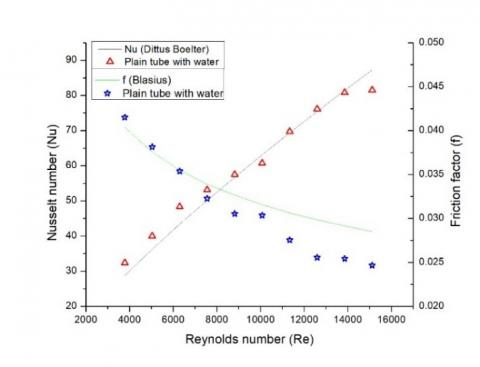Figure 6. Validation of plain tube with the experimental data

6.2 Heat transfer and friction factor results

The heat transfer rate for PATT of different twist ratio (TR)=3, 4 and 5 is shown in the Figure 9. and it can be clearly seen that the Nusselt number is increasing with a decrease in TR. The heat transfer improvement observed is around 1 % - 9.59 % in the TT with TR=3 than that of the plain tube with nanofluid. The Nusselt number enhancement for perforated TT (PTT) and perforated TT with alternate axis (PATT) is more when compared to the regular TT as demonstrated in the Figure 7. The heat transfer increase of PTT at a TR=3 is around 1 % - 9.3 % when compared to regular TT and is 1 % - 18 % than that of the plain tube with nanofluid.  Similarly, the heat transfer augmentation of PATT at a TR=3 is around 9.69 % - 16.13 % when compared to regular TT and is 9.7 % - 19.51 % than that of the plain tube with nanofluid. This increase in PTT and PATT can be attributed to the swirl flow due to the TT, high turbulence created due to the holes in TT for perforated TT and a change in the swirl direction periodically at each alternate axis for PATT.

Likewise, the friction factor observed is around 1.29 % - 22.5 % in the TT with TR=3 than that of the plain tube with nanofluid. The maximum friction factor enhancement for PTT and PATT is slightly more when compared to the regular TT. The friction factor in PTT at a TR=3 is around 1 % - 2 % more when compared to regular TT and is 1 % - 24 % more than that of the plain tube as shown in the Figure 8. Similarly, the friction factor in PATT at a TR=3 is around 1 % - 8.31 % more when compared to regular TT and is 1 % - 28.9 % more than that of the plain tube with nanofluid as demonstrated in the Figure 8. The friction factor for PATT of different twist ratio (TR)=3, 4 and 5 is shown in the Figure 10. The friction factor increase is observed in PTT and PATT as it offers flow resistance with greater pressure drop when compared to regular TT and that of the plain tube.

The fitted values of experimental data of PATT at different twist ratios in a plain tube with SiC nanofluid as in equations (6) & (7) are within ±1.5 % and ±2.28 % for Nusselt number and friction factor respectively.

$N u=0.376 R e^{0.532} P r^{0.415} T R^{-0.196}$ (6)

$f=26.378 R e^{-0.73} T R^{-0.013}$ (7)

## f7.jpgFigure 7. Effect of different twisted tapes on Nusselt number vs Reynolds number in a plain tube with nanofluid

## f8.jpgFigure 8. Effect of different twisted tapes on friction factor vs Reynolds number in a plain tube with nanofluid

## f9.jpgFigure 9. Effect of twist ratio on Nusselt number vs Reynolds number in a plain tube with nanofluid

## f10.jpg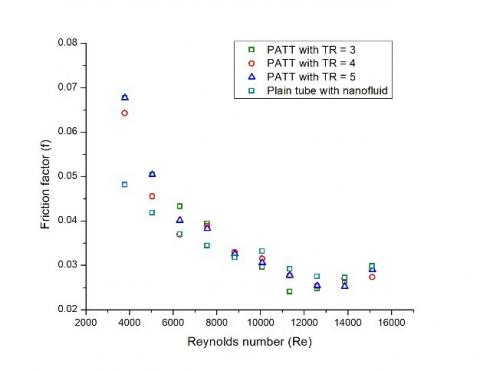Figure 10. Effect of twist ratio on friction factor vs Reynolds number in a plain tube with nanofluid

Comparison between the Nusselt numbers and the friction factors obtained from the present experimental data with those calculated by the present regression equations of water and PATT are portrayed in Figures 11 & 12.

## f11.png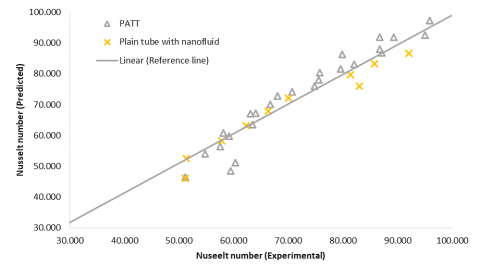Figure 11. Comparison between experimental and predicted values for Nusselt number

## f12.pngFigure 12. Comparison between experimental and predicted values for Friction factor

7. Conclusions

The heat transfer rate and friction factor are analysed and compared for PATT, PTT and TT in a plain tube with nanofluid at 0.1 wt.% concentration containing SiC nanoparticles obtained after 5 h of ball milling at Reynolds number ranges between 3000 and 16000. The results obtained are summarized as below:

•    The heat transfer rate and friction factor are more for PATT, PTT and TT in the decreasing order when compared with the plain tube with nanofluid.

•    As the twist ratio decreases for PATT, the heat transfer rate and friction factor increase.

•    The maximum heat transfer increase for PATT, PTT and TT at a TR of 3 is 19.51 %, 18 % and 9.59 % respectively, when compared to that of the plain tube with nanofluid. Similarly, the maximum friction factor increases for PATT, PTT and TT at a TR of 3 is 28.9 %, 24 % and 22.5 % respectively, compared to that of the plain tube. Hence, the heat transfer rate and friction factor are highest for PATT when compared to PTT and TT.

•    The heat transfer enhancement is seen to be high in case of PATT, hence the regression equations are established for the Nusselt number and friction factor in case of SiC nanofluid flowing through the plain tube inserted with PATT and the attained equations are fitted with the experimental data.

•    Hence, the inserts installed in the plain tube with the nanofluid flowing through it will save more energy when compared to the plain tube with water flowing through it.

Acknowledgment

The financial support given by TEQIP-II through Ministry of Human Resource and Development (MHRD), Government of India for the research project is highly appreciated.

Nomenclature
 h Heat transfer coefficient, W.m-2. K-1 $T_{B}$ Bulk temperature, K $T_{S}$ Mean wall temperature, K $T_{i}$ Inlet temperature of the circular tube, K $T_{o}$ Outlet temperature of the circular tube, K D Diameter of the tube, m L Length of the tube, m m Mass flow rate of the fluid, kg. s-1 U Mean flow velocity of the fluid, m.s-2 cp Specific heat, J. kg-1. K-1 $\Delta p$ Pressure drop (bar) Nu Nusselt number f Friction factor Re Reynolds number Pr Prandtl number Greek symbols Ω Ohms ρ density of fluid, kg. m-3
References

 Yu, W.H., France, D.M., Smith D.S., Singh, D., Timofeeva, E.V., Routbort, J.L. (2009). Heat transfer to a silicon carbide/water nanofluid. International Journal Heat Mass Transfer, 52: 3606–12. https://doi.org/10.1016/j.ijheatmasstransfer.2009.02.036

 Timofeeva, E.V., Smith, D.S., Yu, W., France, D.M., Singh, D., Routbort, J.L. (2010). Particle size and interfacial effects on thermo-physical and heat transfer characteristics of water-based α-SiC nanofluids. Nanotechnology, 21(21): 215703-10. http://dx.doi.org/10.1088/0957-4484/21/21/215703

 Timofeeva, E.V., Yu W., France, D.M., Singh, D., Routbort, J.L. (2011). Base fluid and temperature effects on the heat transfer characteristics of SiC in ethylene glycol/H2O and H2O nanofluids. Journal of Applied Physics, 109(1): 014914. https://doi.org/10.1063/1.3524274

 Nikkam, N., Haghighi, E.B., Saleemi, M., Behi, M., Khodabandeh, R., Muhammed, M., Palm, B., Toprak, M.S. (2014). Experimental study on preparation and base liquid effect on thermo-physical and heat transport characteristics of α-SiC nanofluids. Int. Comm. In Heat and Mass Transfer, 55: 38-44. https://doi.org/10.1016/j.icheatmasstransfer.2014.04.011

 Farhadi, M., Hasanpour, A., Sedighi, K. (2014). A review study on twisted tape inserts on turbulent flow heat exchangers-The overall enhancement ratio criteria. International Communications in Heat and Mass Transfer, 55: 53–62. https://doi.org/10.1016/j.icheatmasstransfer.2014.04.008

 Shabanian, S.R., Rahimi, M., Shahhosseini, M., Alsairafi, A.A. (2011). CFD and experimental studies on heat transfer enhancement in an air cooler equipped with different tube inserts. International Comm. in Heat and Mass Transfer (ICHMT), 38: 383–390. https://doi.org/10.1016/j.icheatmasstransfer.2010.12.015

 Eiamsa-ard, S. (2010). Study on thermal and fluid flow characteristics in turbulent channel flows with multiple twisted tape vortex generators. International Communications in Heat and Mass Transfer, 31: 644–651. https://doi.org/10.1016/j.icheatmasstransfer.2010.02.004

 Eiamsa-ard, S., Thianpong, C., Eiamsa-ard, P., Promvonge, P. (2010). Thermal characteristics in a heat exchanger tube fitted with dual twisted tape elements in tandem. International Communications in Heat and Mass Transfer, 37: 39–46. https://doi.org/10.1016/j.icheatmasstransfer.2009.08.010

 Eiamsa-ard, S., Wongcharee, K., Eiamsa-ard, P., Thianpong, C. (2010). Heat transfer enhancement in a tube using delta-winglet twisted tape inserts. Applied Thermal Engineering, 30: 310–318. https://doi.org/10.1016/j.applthermaleng.2009.09.006

 Eiamsa-ard, S., Thianpong, C., Eiamsa-ard, P., Promvonge, P. (2009). Convective heat transfer in a circular tube with short-length twisted tape insert. International Communications in Heat and Mass Transfer, 36: 365–371. https://doi.org/10.1016/j.icheatmasstransfer.2009.01.006

 Eiamsa-ard, S., Wongcharee, K., Eiamsa-ard, P., Thianpong, C. (2010). Thermohydraulic investigation of turbulent flow through a round tube equipped with twisted tapes consisting of centre wings and alternate-axes. Experimental Thermal and Fluid Science (ETFS), 34: 1151–1161. https://doi.org/10.1016/j.expthermflusci.2010.04.004

 Eiamsa-ard, S., Promvonge, P. (2010). Thermal characteristics in round tube fitted with serrated twisted tape. Applied Thermal Engineering, 30: 1673-1682. https://doi.org/10.1016/j.applthermaleng.2010.03.026

 Eiamsa-ard, S., Seemawute, P., Wongcharee, K. (2010). Influences of peripherally-cut twisted tape insert on heat transfer and thermal performance characteristics in laminar and turbulent tube flows. Experimental Thermal and Fluid Science, 34: 711–719. https://doi.org/10.1016/j.expthermflusci.2009.12.013

 Eiamsa-ard, S., Seemawute, P. (2010). Thermohydraulics of turbulent flow through a round tube by a peripherally-cut twisted tape with an alternate axis. International Communications in Heat and Mass Transfer, 37: 652–659. https://doi.org/10.1016/j.expthermflusci.2009.12.013

 Eiamsa-ard, S., Wongcharee, K. (2011). Friction and heat transfer characteristics of laminar swirl flow through the round tubes inserted with alternate clockwise and counter-clockwise twisted-tapes. International Communications in Heat and Mass Transfer, 38: 348–352. https://doi.org/10.1016/j.icheatmasstransfer.2010.12.007

 Durga Prasad, P.V., Gupta, A.V.S.S.K.S., Deepak, K. (2015). Investigation of trapezoidal-cut twisted tape insert in a double pipe U-tube heat exchanger using Al2O3/water nanofluid. Procedia Materials Science, 10: 50-63. https://doi.org/10.1016/j.mspro.2015.06.025

 Abro, K.A., Chandio, A.D., Abro, I.A., Khan, I. (2018). Dual thermal analysis of magnetohydrodynamic flow of nanofluids via modern approaches of Caputo–Fabrizio and Atangana–Baleanu fractional derivatives embedded in porous medium. Journal of Thermal Analysis and Calorimetry, 135(4): 1-11. https://doi.org/10.1007/s10973-018-7302-z

 Abro, K.A., Rashidi, M.M., Khan, I., Abro, I.A., Tassaddiq, A. (2018). Analysis of Stokes' second problem for nanofluids using modern approach of Atangana-Baleanu fractional derivative. Journal of Nanofluids, 7(4): 738-747(10). https://doi.org/10.1166/jon.2018.1486

 Abro, K.A., Khan, I., Tassaddiq, A. (2018). Application of Atangana-Baleanu fractional derivative to convection flow of MHD Maxwell fluid in a porous medium over a vertical plate. Mathematical Modelling of Natural Phenamenon, 13(1). https://doi.org/10.1051/mmnp/2018007

 Abro, K.A., Hussain, M., Baig, M.M. (2017). An analytic study of molybdenum disulfide nanofluids using the modern approach of Atangana-Baleanu fractional derivatives. The European Physical Journal Plus, 132: 439. https://doi.org/10.1140/epjp/i2017-11689-y

 Sneha, P., Subrahmanyam, T., Naidu, S.V. (2019). A comparative study on the thermal performance of water in a circular tube with twisted tapes, perforated twisted tapes and perforated twisted tapes with alternate axis. International Journal of Thermal Sciences, 136: 530-538. https://doi.org/10.1016/j.ijthermalsci.2018.11.008

 Sneha, P., Subrahmanyam, T., Naidu, S.V. (2019). An experimental investigation on heat transfer and friction factor of Silicon Carbide/water nanofluids in a circular tube. Energy Procedia, 158: 5156-5161. https://doi.org/10.1016/j.egypro.2019.01.682